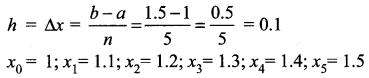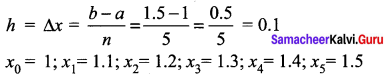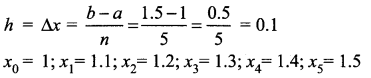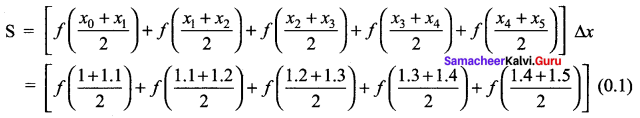# Samacheer Kalvi 12th Maths Solutions Chapter 9 Applications of Integration Ex 9.1

## Tamilnadu Samacheer Kalvi 12th Maths Solutions Chapter 9 Applications of Integration Ex 9.1

Question 1.
Find an approximate value of $$\int_{1}^{1.5} x d x$$ by applying the left-end rule with the partition {1.1, 1.2, 1.3, 1.4, 1.5}
Solution:
Here a = 1, b = 1.5, n = 5, f(x) = x
So, the width of each subinterval isThe left hand rule for Riemann sum,
S = [f(x0) + f(x1)) + f(x2) + f(x3) + f(x4)] ∆x
= [f(1) + f(1.1) + f(1.2) + f(1.3) + f(1.4)] (0.1)
= [1 + 1.1 + 1.2 + 1.3 + 1.4] (0.1)
=  (0.1)
= 0.6.Question 2.
Find an approximate value of $$\int_{1}^{1.5} x^{2} d x$$ by applying the right-end rule with the partition {1.1, 1.2, 1.3, 1.4, 1.5}.
Solution:
Here a = 1;
b = 1.5;
n = 5;
f(x) = x2
So, the width of each subinterval isThe Right hand rule for Riemann sum,
S = [f(x1) +f(x2) +f(x3) + f(x4) + f(x5)] ∆x
= [f(1.1) + f(1.2) + f(1.3) + f(1.4) + f(1.5)] (0.1)
= [1.21 + 1.44 + 1.69 + 1.96 + 2.25] (0.1)
= [8.55] (0.1)
= 0.855.Question 3.
Find an approximate value of $$\int_{1}^{1.5}(2-x) d x$$ by applying the mid-point rule with the partition {1.1, 1.2, 1.3, 1.4, 1.5}.
Solution:
Here a = 1;
b = 1.5;
n = 5;
f(x) = 2 – x
So, the width of each subinterval isThe mid-point rule for Riemann sum,= [f(1.05) + f(1 .15) + f(1.25) +f(1.35) + f(1 .45)] (0.1)
= [0.95 + 0.85 + 0.75 + 0.65 + 0.55] (0.1)
= [3.75] (0.1)
= 0.375.